Home » CALCULATETABLE function DAX

# CALCULATETABLE function DAX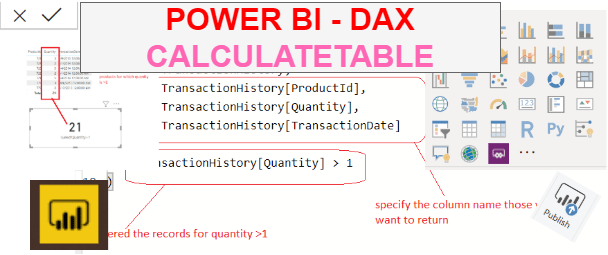CALCULATETABLE function is a power bi filter function in DAX that evaluates a table expression in a context modified by the given filters. It returns a table of values.

SYNTAX

`CALCULATETABLE(<expression>,<filter1>,<filter2>,…)`

<expression> is a table expression to be evaluated. A measure can not be used as expression.

<filter1>,<filter2>.. is a Boolean expression or a table expression that defines a filter.

Lets look at an example of  DAX using CALCULATETABLE  function.

Here we have sample table named TransactionHistory which contains product details as given below.Lets look at an example of using CALCULATETABLE function.

### Basic use of CALCULATETABLE function

Lets create a table named calctable to filter a table records for quantity >1 using following DAX.

```CalCtable = CALCULATETABLE(TransactionHistory,
TransactionHistory[Quantity] >1)```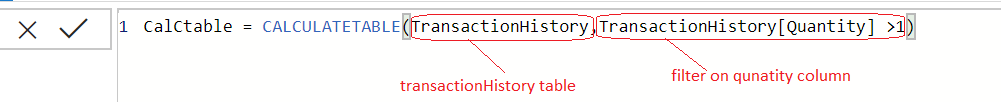Once you commit DAX function, a table named as Calctable is created in data model.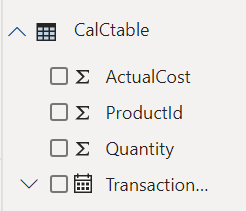This table is just a copy of actual table TransactionHistory but contains only those products for which a quantity value is greater than1.

Lets drag a table fields into a table visual, and you can see all the products having a quantity greater than 1.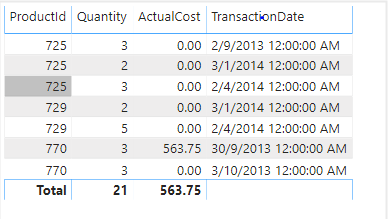You can also compare both tables result sets, you can clearly see that Calctable contains only those products for which quantity value is greater than 1.So, using CALCULATETABLE function you can sort table records based on any specific condition.

### CALULATETABLE function with Summarize function

As you have seen above, a CALCULATETABLE function returns a table based on given filter conditions, It returns all columns of base table, but in case when you want to see only specific columns then this can be done using SUMMARIZE function within CALCULATETABLE function.

Lets modify the above DAX calculation and returns only specific columns ProductID, Quantity, and TransactionDate only.

```CalCtable =
CALCULATETABLE (
SUMMARIZE (
TransactionHistory,
TransactionHistory[ProductId],
TransactionHistory[Quantity],
TransactionHistory[TransactionDate]
),
TransactionHistory[Quantity] > 1
)```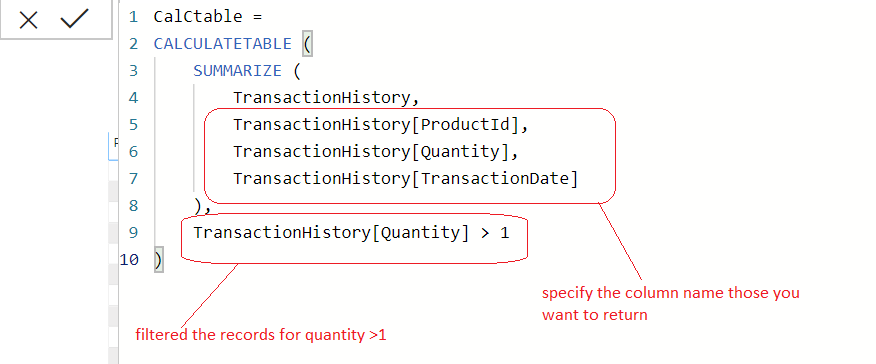Once you commit the DAX, you will get only those columns that you want to see as shown in below screenshot.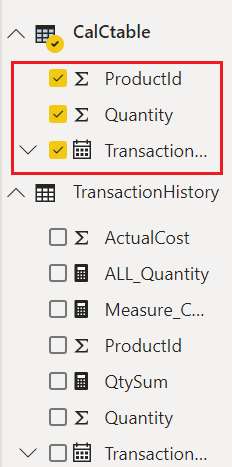### Using CALULATETABLE function within measures

You can also use CALCULATETABLE function within measure based on requirement so rather than creating a separate filtered table it can be used inside any measure.

```SumofQuantity>1 =

SUMX(
CALCULATETABLE (
TransactionHistory,
TransactionHistory[Quantity] > 1
),TransactionHistory[Quantity])```

Now once you commit the DAX, and drag the measure into card visual.

As you can see, it returns a total quantity sum of products for which quantity is greater than1 that is 21 as shown in below screenshot.